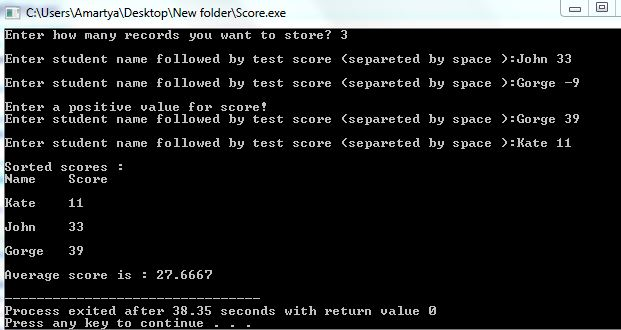# Question & Answer: The program language is C++ for visual studio express 2013…..

The program language is C++ for visual studio express 2013

write a program that dynamically allocates an array that holds a user defined number of names-test scores pairs. The user types a string representing the name of the student, followed by an integer representing that student’s test score. Once the user enters the names and test scores the array should be passed to a function that sorts the test scores in ascending order. Then another function should be called that calculates the average of the test scores. These two functions should take arrays of structures for arguments, with each structure containing the name and score of a single student. Use pointers rather than array indicies. The program should display the sorted scores as well as the average score with appropriate headings. Input validation DO NOT accept negative numbers for test scores.

Don't use plagiarized sources. Get Your Custom Essay on
Question & Answer: The program language is C++ for visual studio express 2013…..
GET AN ESSAY WRITTEN FOR YOU FROM AS LOW AS \$13/PAGE

/*Source code of the above problem is given below:*/

#include<iostream>

#include<cstring>

using namespace std;

struct Student

{

string name;

double score;

};

void sortScore(struct Student *record,int size);

double avgScore(struct Student *record,int size);

int main()

{

int n=0;

string name;

while(n<=0){

cout<<endl<<“Enter how many records you want to store? “;

cin>>n;

if(n<=0)

cout<<endl<<“Enter a positive value!”;

}

Student *record = new Student[n];

for(int i=0;i<n;i++){

double score=-1;

/*name should be a single word and test score should be entered separeted by a space character*/

while(score<0){

cout<<endl<<“Enter student name followed by test score (separeted by space ):”;

getline(cin,name,’ ‘);

(*(record + i)).name = name;

cin>>score;

if(score<0)

cout<<endl<<“Enter a positive value for score!”;

else

(*(record + i)).score = score;

}

}

sortScore(record,n);

cout<<“nSorted scores :”;

cout<<endl<<“Name”<<” “<<“Score”<<endl;

for(int i =0;i<n;i++){

cout<<(*(record + i)).name<<” “<<(*(record + i)).score<<endl;

}

double avg = avgScore(record, n);

cout<<endl<<“Average score is : “<<avg<<endl;

return 0;

}

void sortScore(struct Student *record,int size)

{

//sorting according to scores value

for(int i=0;i<size;i++)

{

for(int j=i+1;j<size;j++)

{

if((*(record + i)).score > (*(record + j)).score)

{

double temp =(*(record + i)).score;

string nameTemp = (*(record + i)).name;

(*(record + i)).score=(*(record + j)).score;

(*(record + i)).name = (*(record + j)).name;

(*(record + j)).score = temp;

(*(record + j)).name = nameTemp;

}

}

}

}

double avgScore(struct Student *record,int size)

{

double sum = 0;

//calculating average of scores

for(int i=0;i<size;i++)

{

sum = sum + (*(record + i)).score;

}

double avg = (double)sum/size;

return avg;

}

/*For better understanding output screenshot is attached here*/